# Detecting Genetic Copynumber with Gaussian Mixture Models

Wednesday, August 7, 2013

Disclaimer: I originally published this post on Counsyl's blog while working at Counsyl, and then reposted it on my own website with their permission for posterity. For the record, Counsyl is an awesome place to work and if you enjoy this sort of stuff - well, they're hiring.

At Counsyl, we test for a variety of autosomal recessive diseases. It turns out that not all genetic diseases are as simple as a single base mutation; some diseases occur as a result of a gene with varying copy number. When a person's chromosomes have enough copies of the gene, they are healthy, but if there are no copies of the gene, complications occur. In order to accurately detect carriers of these types of diseases, we need more than just biological assays — we need reliable algorithms to process the data we get from the biochemical reactions we run and determine the likelihood of carrying the genetic disorder.

## Determining Gene Copy Numbers

Though for some genes single nucleotide polymorphisms (SNPs, as they are commonly known, are changes in a single base of the DNA) are the primary type of mutation to look out for, there are other genes that can and often do undergo copy-number variation. In other words, in addition to watching out for the gene sequence changing, you also have to watch out for the number of copies of the gene present. Some people will simply be missing the gene entirely on one of their chromosomes. Some people will have chromosomes with one copy of the gene, as is normal. And some people will even have two copies of the gene on a single chromosome. For genes that code for some protein, as long as someone has one or more copies, they will be able to produce the protein — but as soon as they're missing the gene entirely, they can no longer produce the protein, which can lead to severe health complications.

Simplifying a bit for the sake of purity and ignoring some gruesome real-world details of biology, what we want to do is detect how many copies of the gene in question someone has. If they have one copy, they're definitely a carrier - at least one of their chromosomes is completely missing the gene. If they have three copies or more, they're almost certainly not a carrier — the probability of having all three copies on the same chromosome is vanishingly small. If they have two copies, they're probabably not a carrier, although there is some ancestry-dependent probability that both copies are on the same chromosome. (This is ancestry-dependent because the concentration of 2-copy chromosomes is different in different human populations, so the probability of having one depends on where your ancestors came from.)

In our lab, we use a reaction called quantitative polymerase chain reaction (qPCR for short) to test for these copy-number variations. The polymerase chain reaction is a method to amplify DNA. Starting with the sample, free nucleotides, short DNA sequences called "primers", and an enzyme called DNA polymerase, PCR amplifies samples exponentially in a number of cycles. In each cycle of PCR, the polymerase will use the spare nucleotides floating around in the solution to make copies of the DNA from the sample, approximately doubling the amount of DNA (the primers are used to get the reaction started). The "quantitative" in qPCR refers to the ability to measure the concentration of DNA in the reaction after every cycle of PCR, rather than just at the end. At the beginning, the concentration grows exponentially (because each cycle approximately results in a doubling of DNA), but after a while the polymerase starts to run out of spare nucleotides, and the concentration growth slows down and eventually reaches a maximum.

And this, finally, is what gets us our answer. Starting with a known concentration of DNA, we can use PCR to amplify only the relevant gene. Let me repeat — the only thing that gets amplified is the piece we're interested in. If the amplification proceeds very slowly, then we can infer that the original amount of the gene was relatively low, and there's probably only one copy of the gene present in the sample. If the amplification proceeds very quickly, then we can infer that there was a large number of gene copies present, so there must be three or more copies. And if the amplification proceeds "just right", then we can infer that there were probably two copies of the gene.

Of course, this is all a ton of handwaving. What is "very quickly"? What is "very slowly", or "just right"? What are we comparing to?

In order to have a point of comparison, we include some known (or "control") samples in our plate of reactions. A useful set of controls will cover the reference normal genotype (2 copies), as well as the possible likely mutations flanking the normal copy number: zero, one, and three copies. These control samples give us a good comparison point, so we can use the amplification data to reliably determine the copy number of the relevant gene in each of the patient samples in the batch.

## From Theory to Practice

Now that we've covered the biological theory behind what we're doing, let's work through how we can really use this data to detect disease carriers.

First of all, let's go ahead and load some sample data (pun thoroughly intended). If you'd like to follow along yourself, we've put up the IPython notebook for this post and the necessary data files on GitHub.

In :
import json

with open(filename, "r") as data_file:
return data

# What's in this file, anyways?
print data.keys()

[u'sample', u'cycles', u'reference']


This file contains a dictionary with three things: cycles, a list of cycle indices, sample, a list of amplification values of the sample we're examining at each cycle, and reference, a list of amplification values for the reference gene at each cycle. We need to include the reference normal genotype in our qPCR in order to have something to compare to: without it, we wouldn't be able to normalize out the effects of varying qPCR efficiency and different initial concentrations of DNA. By comparing to a normal reference, though, we can differentiate between unusual qPCR efficiency and unusual copynumber, since the copynumber would apply only to the sample while the efficiency would affect the reference genes as well.

In :
cycles = data['cycles']
sample_amplification = data['sample']
reference_amplification = data['reference']

print 'First five...'
print 'Cycles:', cycles[0:5]
print 'Sample Amplifications:', sample_amplification[0:5]
print 'Reference Amplifications:', reference_amplification[0:5]

First five...
Cycles: [1, 2, 3, 4, 5]
Sample Amplifications: [-0.005, -0.008, -0.003, 0.001, 0.002]
Reference Amplifications: [-0.002, -0.003, 0.001, 0.001, 0.0]


You'll note that some of the amplification values are negative and all are fractional. The explanation for this is that these are not really the concentration of the DNA, but some other value which is roughly a proxy for how much amplification the DNA has undergone. Since everything we're doing is relative to the controls and references, this doesn't really matter.

(Note that in this case, we have data for every cycle, so cycles is just equal to range(n), but in general this may not be true.)

Let's graph these, and see what we have.

In :
import matplotlib.pyplot as plt

# Display data in three separate plots.
fig, (top, middle, bottom) = plt.subplots(3, 1, sharex=True)
bottom.set_xlabel("Cycle Number", fontsize=13)

ylimits = (-0.5, 3)
for axis in (top, middle, bottom):
axis.set_ylim(ylimits)

# The top plot is the gene amplification data.
top.plot(cycles, sample_amplification, 'ro')
top.set_title("Gene Amplification Data")

# The bottom plot is the reference gene amplification data.
bottom.set_title("Reference Gene Amplification Data")
bottom.plot(cycles, reference_amplification, 'b.', figure=fig)

# The middle plot is both overlaid on top of each other.
middle.set_title("Amplification Data Comparison")
middle.plot(cycles, sample_amplification, 'ro')
middle.plot(cycles, reference_amplification, 'b.')

fig.set_size_inches(5, 8)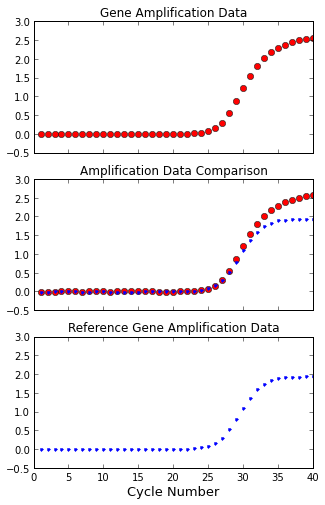As we expected, these are approximately logistic. The amplification starts out exponential, doubling the amount of DNA every cycle, but levels off once the polymerase starts running out of nucleotides to incorporate into new DNA strands or primer to start the DNA synthesis.

Let's fit this data to logistic functions, so we have a function to work with instead of individual data points.

In :
from scipy.optimize import curve_fit
import numpy as np

def logistic(x, vshift, hshift, width, scale):
"""A general logistic curve."""
x = np.asarray(x)
return vshift + scale / (1 + np.exp(-width * (x + hshift)))

def fit_logistic(x, y):
"""Fit a logistic curve to the data."""
# Estimate some initial parameters.
init_vshift = np.min(y)
init_hshift = 30
init_width = 0.01 / np.std(y)
init_scale = np.max(y)

# Fit to our data using a least squares error metric, starting at the initial guesses.
init_params = (init_vshift, init_hshift, init_width, init_scale)
param_opt, param_cov = curve_fit(logistic, np.asarray(x), np.asarray(y), init_params)

return param_opt

# Fit gene in question and reference gene amplification data.
gene_logistic_params = fit_logistic(cycles, sample_amplification)
gene_fit_values = logistic(cycles, *gene_logistic_params)

ref_logistic_params = fit_logistic(cycles, reference_amplification)
ref_fit_values = logistic(cycles, *ref_logistic_params)

# Add the fits to the plots.
top.plot(cycles, gene_fit_values, 'k-')
bottom.plot(cycles, ref_fit_values, 'k-')
middle.plot(cycles, gene_fit_values, 'k-')
middle.plot(cycles, ref_fit_values, 'k-')

display(fig)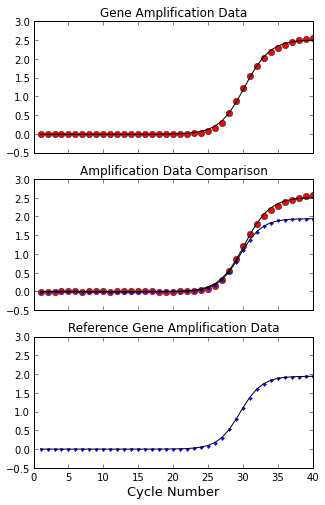Sweet! Given the data for any qPCR reaction, we can fit a function to it and then compute the amplification at any point. But how are we going to use this to determine whether the amplification is going too slow, too fast, or just right for a 2-copy sample?

First, we're going to reduce every sample and reference gene pairing to a single number. We have four reference genes total, and 86 samples, so we're going have 4 * 86 = 344 pairings total, each of which will serve as a data point. Each data point will be represented by just one value, which is going to be the difference in time (measured in cycles) between how long it takes the sample to reach a certain amplification point and how long it takes the reference gene to reach that point.

For clarity, let's go over that again. We're going to choose some value on the y-axis of the graphs above to be our amplification threshold. Then, we're going to use our fitted curves to determine at which cycle the sample reaches that threshold and at which cycle the reference gene reaches that threshold. Finally, each sample-reference pair will be represented by a single value, which is the different of those cycle times. Let's compute this for the example well we've been using.

If our amplification threshold is some value $y$, we'd like to know for which value of $x$ our logistic $f(x)$ is equal to $y$. We've fit values of our parameters $v$, $s$, $w$, and $h$ using curve_fit, so we know the analytical expression for our logistic.

$$f(x) = v + \frac{s}{1 + e^{-w(x + h)}}$$

Therefore, we can pretty easily compute the inverse function, which will get us the $x$ value from the $y$ value.

$$x = - \frac{1}{w} \ln \left(\frac{s}{f(x) - v} - 1\right) - h$$

In :
amplification_threshold = 1.75

def compute_cycle(y_value, vshift, hshift, width, scale):
"""Compute the cycle at which this logistic reaches the ampliciation threshold."""
ct = - (1/width) * log(scale / (y_value - vshift) - 1) - hshift

return ct

gene_ct = compute_cycle(amplification_threshold, *gene_logistic_params)
ref_ct = compute_cycle(amplification_threshold, *ref_logistic_params)

# Draw the threshold in green on the plots.
for axis in (top, middle, bottom):
axis.hlines(amplification_threshold, 0, 40, color='g')

# Draw where the fits hit the threshold.
top.vlines(gene_ct, *ylimits, color='y')
bottom.vlines(ref_ct, *ylimits, color='y')
middle.vlines(gene_ct, *ylimits, color='y')
middle.vlines(ref_ct, *ylimits, color='y')

display(fig)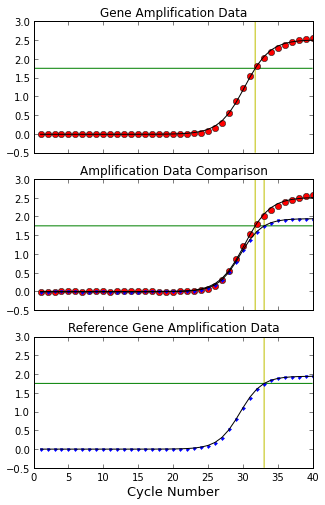Finally, that middle plot is coming in handy! We can see the delta in the cycle value between our gene and the reference gene we're using for this sample. By using these deltas instead of just sample amplification, we've effectively normalized out the effects of the PCR efficiency and initial DNA concentration.

In :
# Compute the delta_ct value for this sample / reference gene pair.
delta_ct = gene_ct - ref_ct
print 'Delta_ct:', delta_ct

Delta_ct: -1.28274003593


Alright. At this point, we've figured out how to take data from our qPCR and compute the delta_ct for each sample and reference pair. Next, we'd like to use these delta_ct values to classify each sample as a 1-copy, 2-copy, or 3-copy sample.

Let's load the delta_ct values for an entire batch.

In :
# Load our delta_ct values for an entire batch.
# What's in this file, anyways?
print data.keys()

[u'RNaseP_replicate1', u'RNaseP_replicate2', u'TERT_replicate2', u'TERT_replicate1']


This file has four parts, one part for each reference gene copy (we have two copies of two different reference genes). The data is separated per replica because the replicas may amplify at different rates, so the same delta_cts for two different replicas actually mean two different things.

We can take a look at what the delta_ct distribution is like for each replica:

In :
def choose_axis(axes, replicate):
"""Choose which subplot to draw on based on the replicate name."""
row = int(replicate[-1:]) - 1

if "TERT" in replicate:
axis = axes[row]
else:
axis = axes[row]

return axis

fig, axes = plt.subplots(2, 2)
for replicate in data:
# Display a histogram of delta_ct values.
axis = choose_axis(axes, replicate)
axis.set_title(replicate + " delta_cts")
axis.hist(data[replicate], bins=30, color='b')

fig.suptitle("delta_ct distributions", fontsize=16);
fig.set_size_inches(10, 10)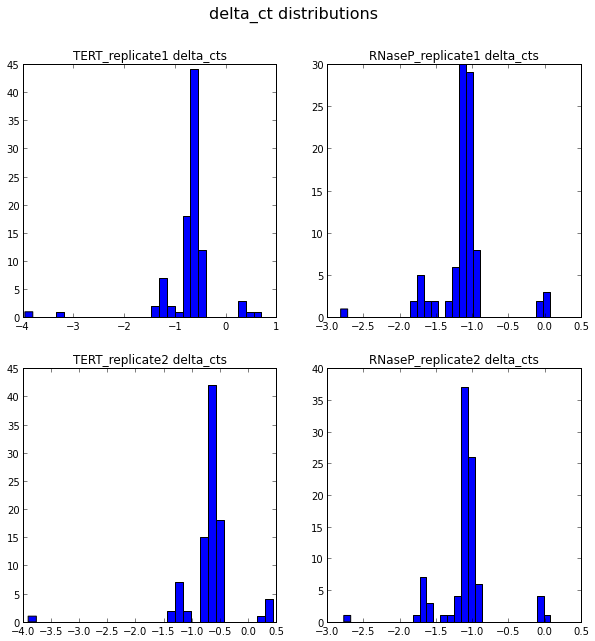We can examine this data visually and see pretty clearly that there's several different clusters, each one with its own peak. This is exactly what we expected! Most samples have two copies of the gene, which is causing the large peak in the middle, as two copies will often produce similar delta_ct values to samples with two copies. On either side of the two copy cluster we see the one and three copy clusters, both of which have a much lower representation because having a deleted or extra gene is a rare mutation.

In order to know precisely where we expect the 1-, 2-, and 3-copy clusters to be, we can use the controls we included. We have two controls of each type, and we can take the mean delta_ct value of those two controls (for each reference gene), and guess that those are approximately at the center of their respective clusters.

Let's take a look at these controls.

In :
# Load the data for the controls.
# What's in this file, anyways?
controls

Out:
{u'RNaseP': {u'1': -0.01150000000000162,
u'2': -1.07275,
u'3': -1.6202499999999995},
u'TERT': {u'1': 0.426499999999999,
u'2': -0.49400000000000066,
u'3': -1.1829999999999998}}

As you can see, for each reference gene we have a delta_ct value for each copy number. Let's see where these lie in the histograms.

In :
def choose_means(controls, replicate):
"""Get the control sample delta_ct means for this reference gene."""
if "TERT" in replicate:
means = controls['TERT']
else:
means = controls['RNaseP']

return {int(copynum_str): val for copynum_str, val in means.items()}

all_lines = []
for replicate in data:
axis = choose_axis(axes, replicate)
means = choose_means(controls, replicate)

# Display the means on the histograms in different colors.
lines = []
for copynumber, color in [(1, 'r'), (2, 'y'), (3, 'g')]:
# Have the vertical lines take up the entire vertical space.
limits = axis.get_ylim()
line = axis.vlines(means[copynumber], *limits, linewidth=4, color=color)
lines.append(line)

# Make sure the vertical lines don't cause rescaling.
axis.set_ylim(*limits)
all_lines.extend(lines)

fig.legend(lines, ("1-copy", "2-copy", "3-copy"), loc=(0.825, 0.75))
display(fig)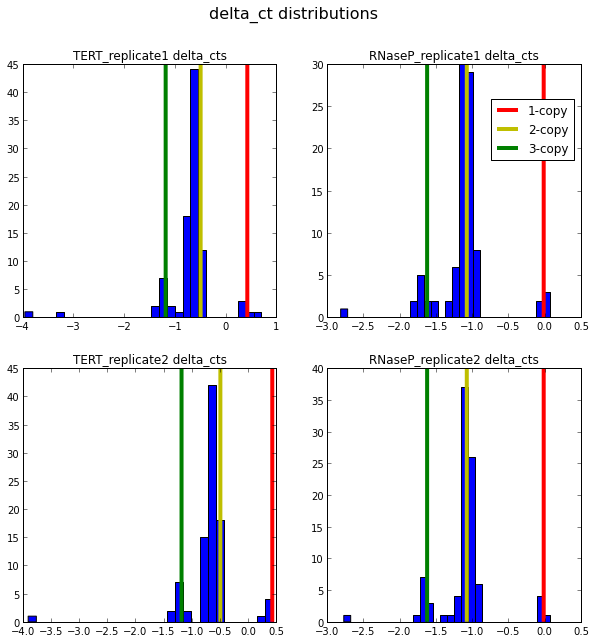Great! We see that the clusters are indeed the different copy numbers, and they're pretty well-separated.

We're almost done — the last step is to assign each sample to one of the three clusters we see, and use those to classify each sample as a 1-copy, 2-copy, or 3-copy sample. We've found that an incredibly robust method of doing that is to fit a Gaussian Mixture Model to these data.

Essentially, we're assuming that our data is coming from a distribution that is composed of three different normal (Gaussian) distributions, each with its own mean and standard deviation. In addition, each normal distribution has a weight, representing how much of the total data is coming from that distribution. Since we have three clusters, that gives us a total of eight parameters: $\mu_1, \mu_2, \mu_3$ for the means of our three clusters, $\sigma_1, \sigma_2, \sigma_3$ for their standard deviations, and finally $w_1, w_2, w_3$ for their respective weights. (That looks like nine parameters, but it's actually only eight, because we constrain the weights such that that $w_1 + w_2 + w_3 = 1$, since each data point must come from one of the three distributions.)

If we knew beforehand which distribution each sample came from, then fitting these parameters would be trivial. But we don't! So in addition to these eight distribution parameters, we need to use our data to figure out which normal component of the mixture model each of the samples came from.

The reason this problem is difficult is that in addition to fitting our label variables (the label of a sample indicates which distribution generated it), we have several unobserved variables that are responsible for generating our labels. (The unobserved variables are the $\mu$s, $\sigma$s, and $w$s, since we have no way of sampling them directly.) If we just had one set of variables, this would be much simpler, but the fact that we have to distinct ones makes this tricky. But we're in luck — there is a standard statistical method known as expectation maximization for solving these sorts of problems.

Expectation Maximization (EM) is an algorithm which starts out with initial guesses for the latent (unobserved) parameters of the distribution, and then iteratively improves them. During the expectation step, we can use our current Gaussian parameters estimates to classify each sample into one of the distributions. During the maximization step, we adjust our Gaussian parameter values based on the current sample labels. We can repeat these two steps several dozen times, and eventually we're guaranteed that the algorithm will converge to a (local) maximum of the likelihood. That is, it'll find a set of parameters and labels that are self-consistent.

Although the final results of our EM algorithm can depend pretty heavily on our initial parameter guesses, our control samples give us a very reasonable starting point, since their copynumbers and delta_ct are known and are likely to be near their respective clusters. With that in mind, let's go ahead and implement our mixture model. (Where by "implement" I of course mean "use the awesome scikit-learn library"!)

In :
from sklearn import mixture
from scipy.stats import norm

models = {}
for replicate in data:
# Create a initial locations and a guess at the weights.
means = choose_means(controls, replicate)
init_means = np.asarray(means.values()).reshape((3, 1))
init_weights = (0.05, 0.8, 0.15)

# Fit a mixture model using these initial locations.
# We use standard initialization procedures for estimating the cluster variances.
gmm = mixture.GMM(n_components=3, init_params='c')
gmm.means_ = init_means
gmm.weights_ = init_weights
gmm.fit(data[replicate])

models[replicate] = gmm


We can now make predictions for each sample. Since we have four replicates (and thus four predictions), we can only assign a prediction to a sample when all the replicates produce the same result.

In :
# Gather all predictions.
predictions = {}
for replicate, values in data.items():
predictions[replicate] = models[replicate].predict(values)

# Convert this into one tuple per sample.
sample_predictions = zip(*predictions.values())

def assign_prediction(replicate_preds):
"""Only assign a prediction if all replicates agree."""
first_prediction = replicate_preds
all_identical = all(pred == first_prediction for pred in replicate_preds)

if all_identical:
return first_prediction
else:
return None

classifications = map(assign_prediction, sample_predictions)


That's it! We now have a classification for each sample. Each classification has been checked four times, once per replicate, and only classified if all of the replicates agree. That may seem like a pretty harsh condition, but we can check that it's working nonetheless.

In :
# Recall that the 0-th component is the 1-copy samples.
print '1-copy samples:', classifications.count(0)
print '2-copy samples:', classifications.count(1)
print '3-copy samples:', classifications.count(2)
print
print 'Failed to classify:', classifications.count(None)

1-copy samples: 5
2-copy samples: 75
3-copy samples: 12

Failed to classify: 0


Let's also take a look to see what our distribution of classifications is.

In :
def recolor(counts, bins, batches, values, classifications):
"""Recolor a histogram according to the sample classifications."""

for patch, right, left in zip(patches, bins[1:], bins[:-1]):
# Get sample classifications, and count how many are in each class.
classes = np.extract(np.logical_and(values >= left, values <= right), np.asarray(classifications))
class_counts = [np.count_nonzero(classes == cls) for cls in [0, 1, 2, None]]

# Find out which class is the plurality, and choose the color based on that.
plurality = class_counts.index(max(class_counts))
color = ['r', 'y', 'g'][plurality] if plurality is not None else 'k'
patch.set_facecolor(color)

fig, axes = plt.subplots(2, 2)
for replicate in data:
# Display a histogram of delta_ct values.
axis = choose_axis(axes, replicate)
axis.set_title(replicate + " delta_cts")
counts, bins, patches = axis.hist(data[replicate], bins=30)
recolor(counts, bins, patches, np.asarray(data[replicate]), classifications)

fig.suptitle("labeled delta_ct distributions", fontsize=16);
fig.legend(lines, ("1-copy", "2-copy", "3-copy"), loc=(0.825, 0.75))
fig.set_size_inches(10, 10)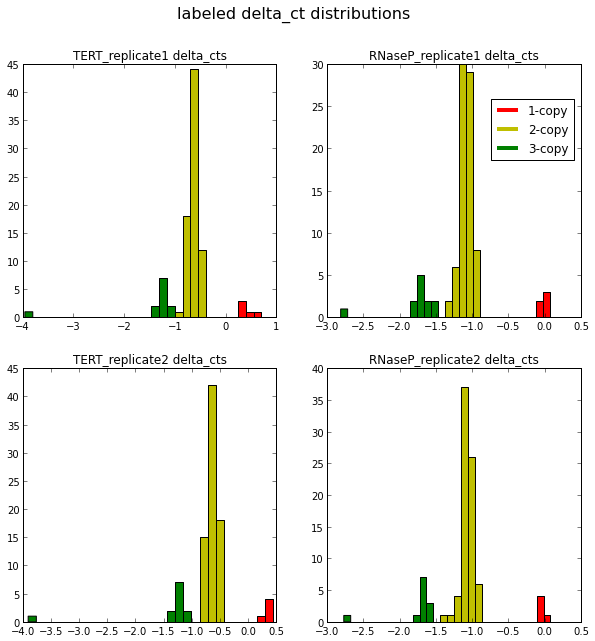And that's that. We've developed a complete algorithm to bring us from raw data in the form of qPCR output to deciding whether each sample has one, two, or three copies of the disease-preventing gene. In practice, this method is very robust and quick, and manages to classify the majority of samples. Though we use several improvements to make this even better, the fundamental ideas behind copy number detection are the same. Samples that are not classified or that are classified as carriers can be retested multiple times to ensure correctness, and of course all results are ultimately vetted by our professional wetlab staff before being reported to our customers.